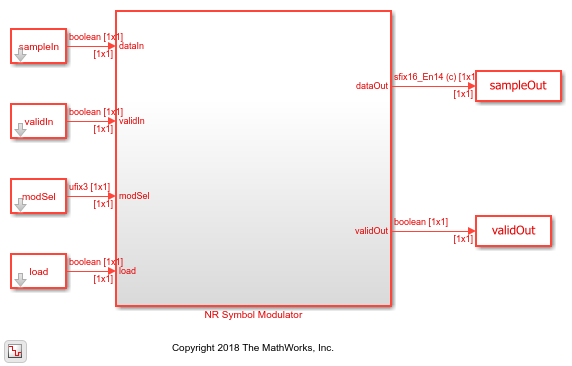# NR Symbol Modulation of Data Bits

This example shows how to use the NR Symbol Modulator block to modulate data bits to complex data symbols. You can generate HDL code from this block.

Set up input data parameters. Choose a data length for each modulation type. The data length must be an integer multiple of number of bits per symbol.

```rng(0); framesize = 240; % Map modulation names to values % 0 - BPSK % 1 - QPSK % 2 - 16-QAM % 3 - 64-QAM % 4 - 256-QAM % 5 - pi/2-BPSK % others - QPSK % for NR Symbol Modulator Simulink block modSelVal = [0;1;2;3;4;5]; % for nrSymbolModulate function modSelStr = {'BPSK','QPSK','16QAM','64QAM','256QAM','pi/2-BPSk'}; outWordLength = 16; numframes = length(modSelVal); dataBits = cell(1,numframes); modSelTmp = cell(1,numframes); nrFcnOutput = cell(1,numframes); ```

Generate frames of random input samples.

```for ii = 1:numframes dataBits{ii} = logical(randi([0 1],framesize,1)); modSelTmp{ii} = fi(modSelVal(ii)*ones(framesize,1),0,3,0); end ```

Convert the framed input data to a stream of samples and input the stream to the Simulink block.

```idlecyclesbetweensamples = 0; idlecyclesbetweenframes = 0; [sampleIn, ctrl] = whdlFramesToSamples(dataBits,idlecyclesbetweensamples,... idlecyclesbetweenframes); [modSel, ~] = whdlFramesToSamples(modSelTmp,idlecyclesbetweensamples,... idlecyclesbetweenframes); load = logical(ctrl(:,1)'); validIn = logical(ctrl(:,3)'); sampletime = 1; samplesizeIn = 1; simTime = size(ctrl,1); ```

```modelname = 'nrhdlSymbolModulatorModel'; open_system(modelname); sim(modelname); ```Export the stream of modulated samples from Simulink to the MATLAB workspace.

```sampleOut = squeeze(sampleOut).'; nrHDLOutput = sampleOut(squeeze(validOut)); ```

Modulate frame data bits with nrSymbolModulate function and use the output of this function as a reference data.

```for ii = 1:numframes nrFcnOutput{ii} = nrSymbolModulate(dataBits{ii},modSelStr{ii}).'; end ```

Compare the output of the Simulink model against the output of nrSymbolModulate function.

```fprintf('\nNR Symbol Modulator\n'); nrFcnOutput = fi(cell2mat(nrFcnOutput),1,outWordLength,outWordLength-2); error = sum(abs(nrHDLOutput-nrFcnOutput(1:length(nrHDLOutput)))); fprintf('\nTotal number of samples differed between Behavioral and HDL simulation: %d \n',error); ```
```NR Symbol Modulator Total number of samples differed between Behavioral and HDL simulation: 0 ```